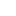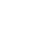# Determination of heat of solution of Potassium

Determination of heat of solution of Potassium Chloride by calorimetric method

#### Theory:

By using calorimeter the heat of solution of potassium chloride can be measured. The instrument which is used to measure the heat of solution of soluble substances is known as calorimeter. The heat of solution of a salt in water may be determined by dissolving it in a known amount of solvent. Change in temperature shows the heat of solution.

#### Heat of solution of KCL:

Heat of solution can be defined as

“The amount of heat released or absorbed when a certain amount of solute is dissolved in a certain amount of solvent” is known as heat of solution. In other words the heat changes occur when 1 mole of solute is dissolved in one liter of solvent.

Heat change may be

• Endothermic
• Exothermic

Heat absorbed or released by a substance during a change in temperature depends upon specific heat. As a result of mass of substance dissolved in a solvent and temperature changes occur.

q = mCP∆T

Where

q = heat of solution

m = mass of solute

CP = specific heat

∆T = change in temperature (Tf-Ti)

Usually when known amount of potassium chloride is dissolved in known amount of distilled water then ions are produced

KCl → K+ + Cl

Polar water molecules are attached by the charge on potassium and chloride ion. KCl absorbs heat from its surrounding when it is dissolved in water. Therefore the dissolution of KCl is an endothermic reaction and which is shown by positive sign (+). Due to change in temperature, this is dropped from water and into the salt. This heat of solution can be determined

#### Apparatus:

• Calorimeter
• Thermometer
• Beakers
• Stirrer
• Tripod stand
• Wire gauze
• Electrical balance

#### Chemicals required:

• Potassium chloride
• Distilled water
• Tap water

#### Procedure:

• First of all required glassware was washed clearly and deride properly
• Then empty calorimeter was weighed with the help of electrical balance
• After that 50 ml of distilled water was taken in calorimeter and temperature of which was determined in the help of thermometer for 5 minutes after every 30 second interval five readings were taken, which was the temperature of cold water
• Then weight of 0.37 gram KCL was added in the water present in calorimeter
• With constant stirring, the change in temperature was noted after every 30 second until the temperature became constant
• Then weight of solution was determined with the help of electrical balance by weighing the calorimeter cylinder
• Molarity of KCL was calculated by using the amount of substance given in experiment
• Enthalpy of solution of KCL was calculated by using the formula

∆Hn = WCPx × ∆T + Cp∆T / m

#### Observations and calculations:

Given mass of KCl = 0.37 g

Molar mass of KCl = 73.5 g/ mol

Molality = mass of solute ×1000/ molar mass

×weight of solution

Molality = 0.37g × 1000/ 73.5× 49.15

Molality = 0.1 m

Determination of heat of solution of Potassium Chloride by calorimetric method

 Time (second) Temperature of cold water Temperature of solution 0 16 30 16 60 16 90 16 120 16 150 16 180 16 210 15.9 240 15.6 270 15.3 300 15.3

So

∆T = Tcold – Tsolution

= 16 – 15.3

= 0.7 oC

Weight of empty calorimeter = 88.46 g

Weight of filled calorimeter = 137.61 g

Weight of solution =137.61 – 88.46

= 49.15g

CPx = 37.57J/mol

Now heat of solution can be calculated as

∆Hn = WCPx × ∆T + Cp∆T / m

∆Hn = 49.15 (37.57) × 0.7 +1 (0.7)/0.1

∆Hn = 12932.9 KJ/mol

#### Result:

Enthalpy of solution of KCL is 12932.9 kilo joule per mole

Determination of heat of solution of Potassium Chloride by calorimetric method###### Don’t miss these Chemistry topics!
Sharing Is Caring:

### 7 thoughts on “Determination of heat of solution of Potassium”

1.Honestly say that I didn’t find such a splendid site ever….. I liked it because of the practicals related to physical chemistry which are complete and accurate…..I will share it with my friends especially with juniors to get knowledge from this amazing platform……. Keep it up I will support you 👏👏👏👏

•thank you

2.Mine is not a comment but a practical question as well.
Heat of solution of KCl.
Vol. Of water= 75ml
Gram of KCl=2g
Temp. of water=30°C
Temp. of solution=29°C
Heat capacity of H2O=4.18
Questions.
1. Calculate how many calories were involved in the dissolution
2. Calculate how many would be involved in 1mol of kcl
3. The ℅ error. Acceptable value given=-8468.68J

3.I love the site and really enjoying the lectures

4.This is the perfect webpage for everyone who wishes to understand this topic. You realize so much its almost hard to argue with you (not that I personally would want toÖHaHa). You definitely put a brand new spin on a subject that has been written about for a long time. Great stuff, just great!

5.6.